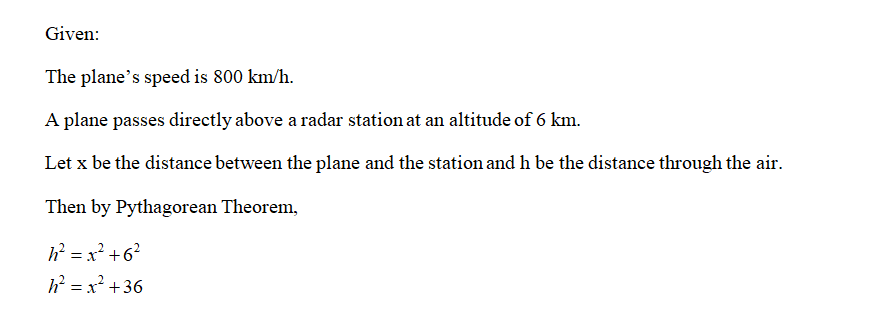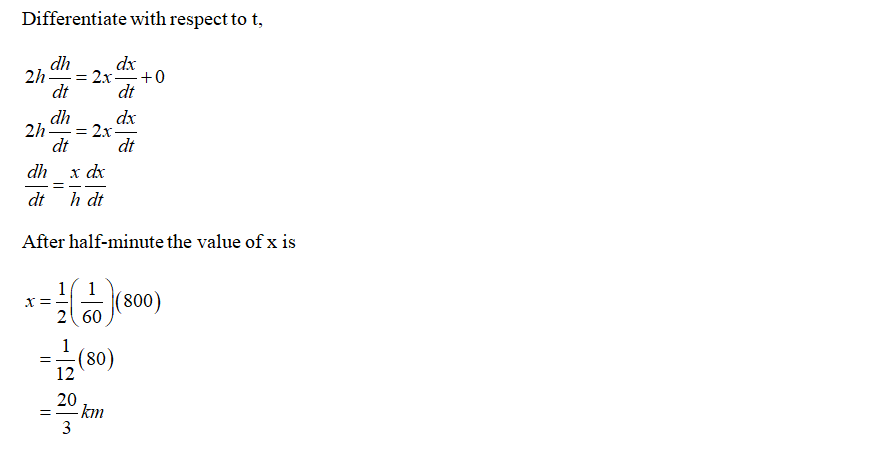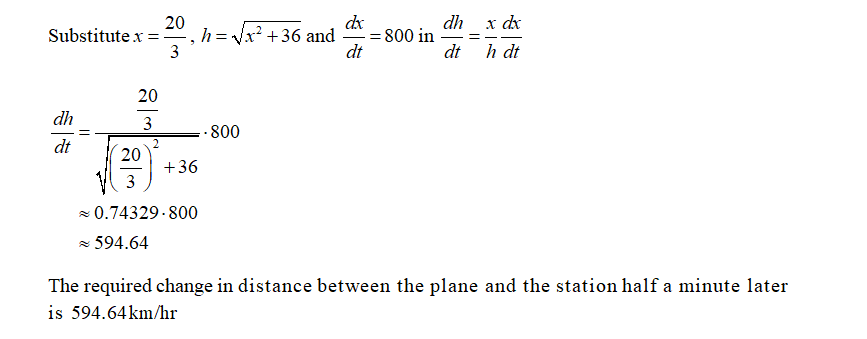# At a given moment, a plane passes directly above a radar station at an altitude of 6 km. (a) The plane’s speed is 800 km/h. How fast is the distance between the plane and the station changing half a minute later? (b) How fast is the distance between the plane and the station changing when the plane passes directly above the station?

Question
1 views

At a given moment, a plane passes directly above a radar station at an altitude of 6 km.
(a) The plane’s speed is 800 km/h. How fast is the distance between the plane and the station changing half
a minute later?
(b) How fast is the distance between the plane and the station changing when the plane passes directly above
the station?

check_circle

Step 1Step 2Step 3...

### Want to see the full answer?

See Solution

#### Want to see this answer and more?

Solutions are written by subject experts who are available 24/7. Questions are typically answered within 1 hour.*

See Solution
*Response times may vary by subject and question.
Tagged in